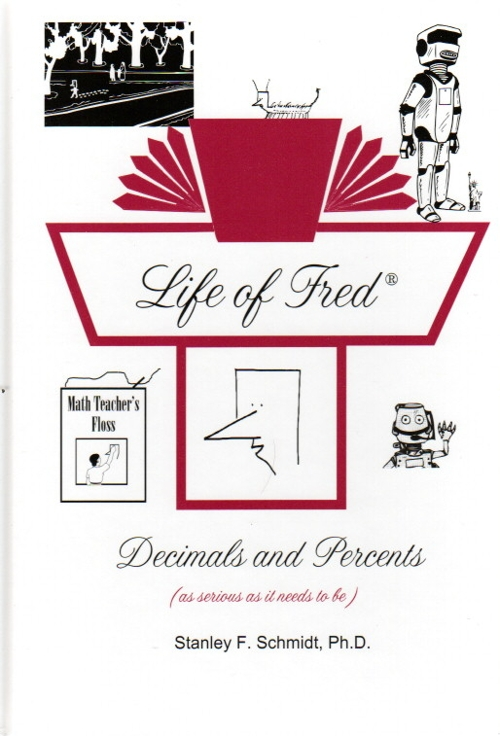Life of Fred Decimals and Percents \$24 : Number Systems, Adding and Subtracting Decimals, Multiplying by Ten, Functions and Inverse Functions, Pi, Multiplying Decimals, Whole Numbers, Squaring a Number, Subtracting Mixed Units, Sets and Subsets, Union and Intersection of Sets, Rules of Divisibility, Division of Decimals, Repeating Decimals, Bar Graphs, Prime and Composite Numbers, Consecutive Numbers, the Goldbach Conjecture, Area of a Circle, Dollars vs. Cents, Pie Charts, Conversion between Percents/ Fractions/ Decimals, 40% of 15, 30% off, Distance Equals Rate Times Time, 15% more, Area of a Triangle, Square Roots, Area of a Parallelogram, 13 Is What Percent of 52, Ratio, Ordered Pairs, Graphing, Negative Numbers, Elapsed Time, Probability. ISBN: 978-0-9791072-0-7, hardback, 192 pages. \$24